# ALPHY5: LIGHT

##### This unit explains light in Physics

Reflection Of Light At A Plane Surface.

Reflection of light:
When a beam of light is incident on a surface, a part of it is returned into the same medium. This phenomenon is called reflection of light.
KINDS OF REFLECTION: There are usually two types of reflection:
Regular reflection Irregular reflection or Diffused reflection
1. Regular reflection occurs when a beam of light falls on a smooth and polished surface.

2. If the incident beam is parallel, the reflected beam is also parallel and in a fixed direction.
E.g., Reflection of light from a plane mirror.
1. Irregular reflection occurs when a beam of light falls on a rough surface.
2. When a parallel beam of light falls on a rough surface, the reflected beam gets scattered in all directions and spreads over a wide area.
E.g., Reflection of light from the wall of a room, newspaper, page of a book or any other object.
[Note: It is the diffused light obtained by reflection from the uneven surfaces which enables us to see the objects around us.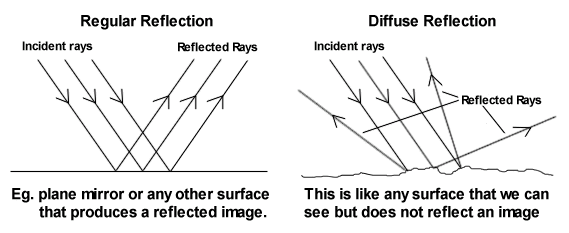1.       Point Of Audience It is the point on the reflecting surface on which the ray of light falls. 2.Incident ray (AO) It is the ray of light that falls on or strikes the reflecting surface. 3.Normal (ON) It is an imaginary line drawn perpendicular to the reflecting surface at the point of incidence. 4.Reflected ray (OB) It is the ray of light that is sent from the reflecting surface back into the same medium in which the incident ray is travelling. 5.Angle of incidence It is the angle formed between the incident ray and the normal. 6.Angle of reflection It is the angle formed between the normal and the reflected ray.

LAWS OF REFLECTION:
The reflection at a surface obeys two laws, which are called the laws of reflection.
1.The angle of incidence is equal to the angle of reflection (i.e., i = r).

2. The incident ray, the reflected ray and the normal at the point of incidence, all lie in the same plane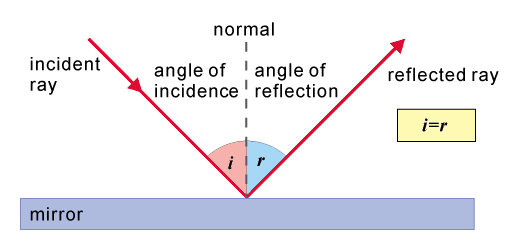Reflection of a ray of light normally incident on a plane mirror

When a ray of light is incident normally on a surface, Angle of incidence, i = 0
Therefore the angle of reflection, r = 0
Thus, a ray of light AO incident normally on a surface is reflected along the same path OA.

FORMATION OF IMAGE BY REFLECTION
1. To find the position of image of an object formed by a mirror after reflection, wALPHY5: LIGHT ASSIGNMENTALPHY5: LIGHT ASSIGNMENTALPHY5: LIGHT ASSIGNMENTALPHY5: LIGHT ASSIGNMENTe need
to consider at least two rays of light incident on the mirror from a point of the object.
2. Each incident ray gets reflected obeying the laws of reflection.
3. The point where the two reflected rays actually meet or they appear to meet (when
produced backwards), gives the position of image of that point of object.

TYPES OF IMAGE:
The image can be of two types –

(i) Real image
(ii) Virtual image

DIFFERENCE BETWEEN A REAL AND VIRTUAL IMAGE

 REAL IMAGE VIRTUAL IMAGE 1. A real image is formed when two or more rays after reflection actually meet at a point. 1. A virtual image is formed when two or more rays after reflection do not actually meet but appear to meet at a point 2. A real image can be obtained on a screen. 2. A virtual image cannot be obtained on a screen 3. A real image is always inverted with respect to the object. 3. A virtual image is always erect with respect to the object.

IMAGE OF A POINT OBJECT FORMED BY A PLANE MIRRORMM1 = A plane mirror O = A point object I = Image of the point object

1. To show the formation of image by a plane mirror, we need at least two rays
starting from the point object O.
2. Let OA and OB be two rays incident on the plane mirror from the point object
O.
3. AN1 and BN2 are the normals at points A and B.
4. The rays OA and OB get reflected from a mirror in directions AC and BD
respectively obeying laws of reflection.
5. When seen from a position between C and D, rays AC and BD appear to come
from some point I behind the mirror.
6. The point I is the image of the object O.

CHARACTERISTICS OF IMAGE FORMED IN A PLANE MIRROR:

1. Plane mirrors are used as looking glass

2. Plane mirrors are also used in constructing periscope which is used in submarines.

3. Plane mirrors are used in solar cookers.

4. Plane mirrors are used to make a kaleidoscope, a toy which produces beautiful patterns from coloured paper,
pieces of glass or small coloured beads.

5. Plane mirrors are also used to create illusions and

Reflection Of Light At Curved Surfaces.

Curved mirrors are parts of a sphere. The following terms are used in curved mirrors:• Pole P– it is the centre of the mirror.
• Centre of curvature C– it is the centre of the sphere of which the mirror is part.
• Radius of curvature r– it is the radius of the sphere of which the mirror is part.
• Principal axis– it is a line drawn through the pole of the mirror and the centre of curvature.
• Principal focus F – for a concave mirror, it is the point at which all rays parallel and close to the principal axis converge at after reflection. In the case of a convex mirror, it is the point at which all rays parallel and close to the principal axis appear to diverge from after reflection. (See the figure above). It is also called the focal point.
• Focal plane– it is a plane perpendicular to the principal axis and passes through the focal point. It is the plane where parallel rays but not parallel to the principal axis converge at or appear to diverge from after reflection.
• Focal length f– it is the distance between the pole of the mirror and its focal point.

When rays are produced behind the mirror, they are indicated using dotted lines. This means that they are imaginary or virtual. Hence the focal point and focal length of a concave mirror are real while the focal point and focal length of a convex mirror are virtual. A real focal length is given a positive sign while a negative focal length is given a negative sign.

Ray diagrams

Curved mirrors form images when two rays intersect or appear to intersect. In ray diagrams, we use the following symbols to represent the two curved mirrors: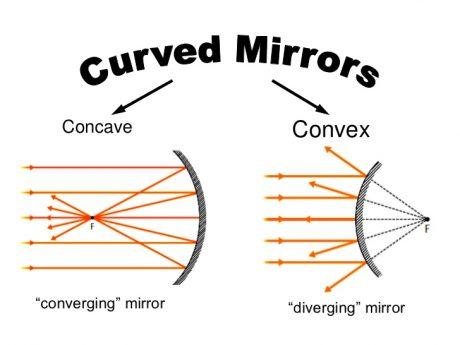There are four important rays used in ray diagrams. They include:

• A ray passing through C or appearing to pass through C:The ray is reflected along the same path.

• A ray parallel and close to the principal axis.

The ray is reflected through the principal focus F for a concave mirror or appear to come from the principal focus of the convex mirror.• A ray passing through the principal focus F or appearing to pass through F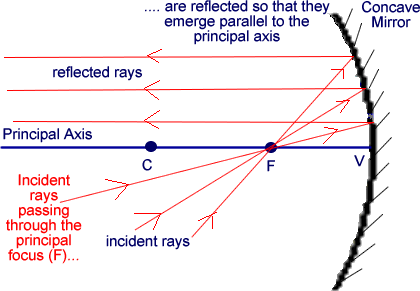The reflected ray moves parallel to the principal axis (by the principle of reversibility of light).

• A ray incident at the pole of the mirror.The ray is reflected making the same angle with the principal axis as the incident ray .

Image formation by curved mirrors

The table below provides a summary of how a concave and convex mirror forms images:

 Position of the object Image formation by concave mirror Image formation by convex mirror Object at infinity Image formed is inverted, real , diminished and formed at F. Image formed is upright, virtual and diminished. Object beyond C Image formed is real, inverted and diminished. Image formed is virtual, upright and diminished. Object at C Image formed is real, inverted and same size as the object Image formed is virtual, upright and diminished. Object between C and F Image formed is real, inverted and magnified. Image formed is virtual, upright and diminished. Object at F Image formed is real, inverted and at infinity. Image formed is virtual, upright and diminished. Object between F and P Image formed is virtual, upright and magnified. Image formed is virtual, upright and diminished.

Note that a concave mirror always forms real and inverted and images except when the object is placed between the focal point and the pole of the mirror when it forms a virtual and inverted image. On the other hand, a convex mirror always forms a virtual, erect and diminished image.

A real image is that image formed by actual intersection of real rays while a virtual image is formed by imaginary rays. Furthermore, a real image can be formed on a screen while a virtual image cannot be formed on a screen.

Linear magnification and the mirror formula

Linear magnification is defined as the ratio of the image size to the object size;

Magnification= Image size/ Object size.

Similarly, it can be expressed as the ratio of the distance of the image to the distance v of object u from the mirror. Magnification has no unit.

Suppose an object is placed u cm in front of a spherical mirror of focal length f such that the image is formed v cm from the mirror, then u, v and f are related by the equation;

1/f= 1/u + 1/v.

This equation is referred to as the mirror formula. The formula holds for both concave and convex mirrors.

When applying the mirror formula, it is necessary to observe the following points:

• That all distances are measured from the mirror as the origin.
• All real distances are positive while all virtual distances are negative.
• A concave mirror has a positive focal length while a convex mirror has a negative focal length.

Determination of the focal length of a concave mirror

The focal length of a concave mirror can be estimated by focusing a distant object on a screen. Parallel rays from a distant object converge at the focal plane of the mirror.The distance between the mirror and the screen is the estimated focal length of the concave mirror.

### Applications of spherical mirrors and curved reflecting surfaces

Concave mirrors are used by dentists to magnify teeth during extraction and as a shaving mirror. In both cases, the mirror forms upright and magnified images. Concave reflectors are also used in projector lamps, solar concentrators and telescopes.Convex mirrors can be used in supermarkets to monitor customers’ activities and as driving mirrors in cars and motorcycles. This is because convex mirrors provide a wide field of view compared to a plane mirror.REFRACTION OF LIGHT THROUGH TRIANGULAR PRISMS.

Prism is a triangular glass, which has two triangular bases and three rectangular lateral surfaces.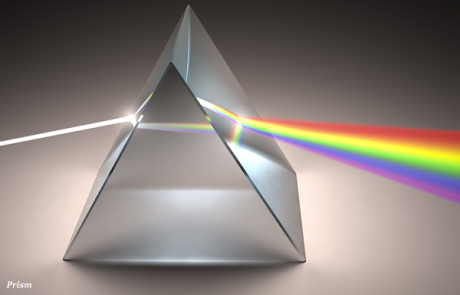• The unique shape of the prism makes the emergent ray bend at an angle to the direction of the incident ray and this angle is known as the angle of deviation.
• The angle between two lateral faces of a prism is known as the angle of the prism.

## Dispersion of White Light by a Glass Prism

• As shown in the image given above, the prism has split the incident white light into a band of colours.
• The various colours those are seen through the prism are arranged in order; this order is named as ‘VIBGYOR.’
• VIBGYOR is constituted after taking the first letter of all the following colours −
• V − Violet
• I − Indigo
• B − Blue
• G − Green
• Y − Yellow
• O − Orange
• R − Red
• The band of the colored components of a light beam is known as spectrum and VIBGYOR is the sequence of colors that you can see in the above image.
• The splitting of light into different colours is known as dispersion.
• All the colours have different bending angles in respect to the incident ray; the red light bends list (can be seen at the top) whereas the violet bends the most (see the image given above).
• Because of having different bending angles, all the colors become distinct.
• Newton was the first scientist who used a glass prism to obtain the spectrum of sunlight and he concluded that the sunlight is made up of seven colors.

## Atmospheric Refraction

• The deviation of light rays from a straight path in the atmosphere (normally because of variation in air density) is known as atmospheric refraction.
• Atmospheric refraction nearby the ground produces mirages, which means, the distance objects appear elevated or lowered, to shimmer or ripple, stretched or shortened, etc.
• In the night, the stars appear twinkling, it is also because of the atmospheric refraction.
• Because of the atmospheric refraction, the Sun remains visible and about 2 minutes after the actual sunset and about 2 minutes before the actual sunrise (see the image given below).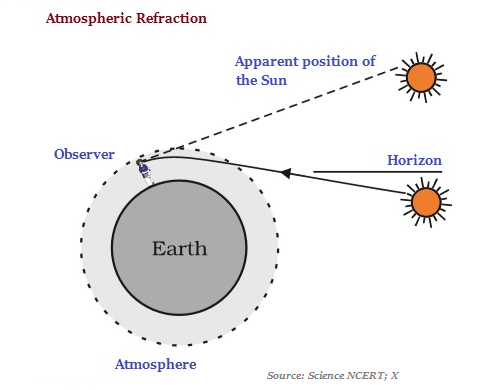## Tyndall Effect

• The earth’s atmosphere is largely constituted of a heterogeneous mixture, such as tiny water droplets, suspended particles of dust, smoke, and molecules of air. When a beam of light strikes through such fine particles, the path of the beam gets scattered. The phenomenon of scattering of light by the colloidal particles (of the atmosphere) gives rise to Tyndall effect.
• The scattering of light makes the particles visible in the atmosphere.
• Very fine particles scatter largely blue light whereas particles of larger size scatter the light, which has longer wavelengths.
• The red light has a wavelength (about) 1.8 times larger than the blue light.

Refraction Of Light Through A Thin Lens.

Lenses form images by refraction of incident light.  Most commonly they are constructed of glass, although other transparent materials are also used.  Similar to spherical mirrors, there are two basic types of lens, converging and diverging .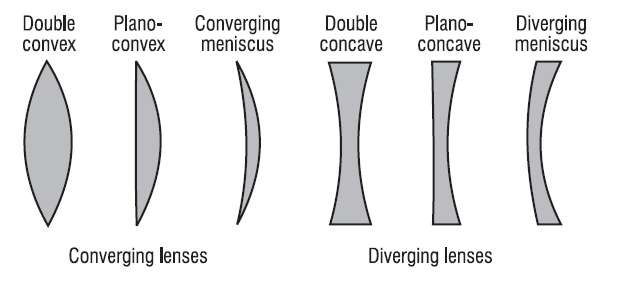Converging Lens.  Parallel light incident on one side of a converging lens passes through a single point on the other side called the “focal point” (F).  This is achieved due to refraction at both surfaces of the lens because light travels more slowly in glass than air.Diverging Lens.  Parallel light incident on one side of a diverging lens is seen to diverge, as if the light came from a single point on the same side of the lens as the incident light.  This point is known as a “ virtual focal point“, since after passage through the lens, light does not pass through it. As in the case of the converging lens light is refracted at both surfaces passing more slowly through glass than air.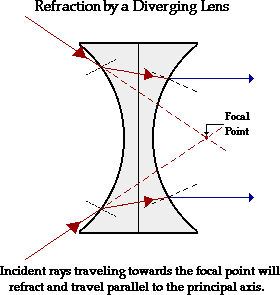Lens Makers Formula.  The focal length of a lens depends on the refractive index of the material of the lens (n L ), the refractive index of the medium in which it is immersed (n m ) and the radii of curvature of the two faces of the lens (R 1 and R 2 ) according to the   formula (valid for thin lenses),Whether a surface is convex or concave is determined by light striking the lens before passing through the lens.  For example, the first surface struck by the light in the diagram of the diverging lens above is concave.  If this convention is adhered to it does not matter which surface is labeled R1 or R 2.
• Image Formation:  Similar to the spherical mirror case there are three characteristic rays, any two of which enable the location of the image to be determined.
• Rays passing through the centre of a lens are transmitted without deflection.
• Rays parallel to the axis are refracted such that they pass through the focal point of the lens (converging) or appear to have come from the focal point (diverging).
• Rays passing through the focal point are then refracted parallel to the axis of the lens (converging) or rays which are headed towards the focal point on the other side of the lens are refracted parallel to the exis (diverging).

Converging Lens:  Examples of image formation using the characteristic rays are shown below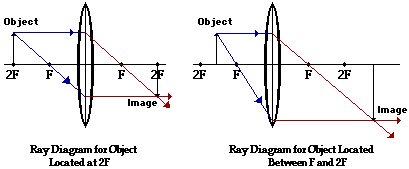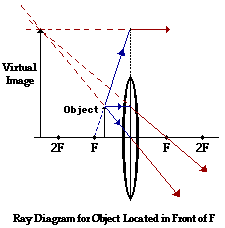Diverging Lens:  Example of image formation using the characteristic rays is shown below.Optical Instruments

Optical instruments are the devices which process light wave to enhance an image for more clear view. Use of optical instruments, such as a magnifying lens or any complicated device like microscope or telescope usually makes things bigger and helps us to see in a more detailed manner. The use of converging lenses makes things appear larger and on the other hand, diverging lenses always gets you smaller images.

While using a converging lens, it’s important to remember that, if an object is at a larger distance then the image is diminished and will be very nearer to the focal point. While the object keeps on moving in the direction of the lens, the image moves beyond the focal point and enlarges. When object is placed at 2F, which is two times the focal distance from the lens, the image and object becomes of the same size. When the object moves from 2F towards the focal point (F), it’s image keeps moving out of the lens and enlarges till it goes to infinity when the object reaches the focal point, F. As the object moves closer to the lens, the image moves in the direction of the lens from negative infinity and gets smaller when the object gets closer to lens.

Telescopes

A basic refracting telescope is an optical instrument that has two optical elements, an objective and an eyepiece.  We have two thin lenses in air.  The objective is a large lens that collects light from a distant object and creates an image in the focal plane, which is a faithful representation of the object.  The eyepiece is a sophisticated magnifying glass through which we view this image.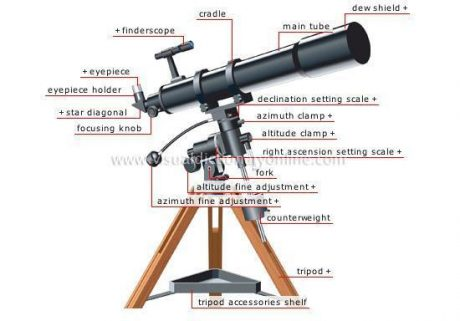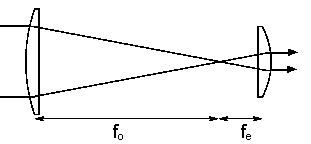Keplerian Telescop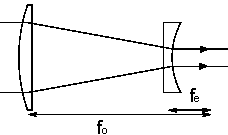Galilean Telescope

A Keplerian telescope has a converging lens eyepiece and a Galilean telescope has a diverging lens eyepiece.  The distance between the image and the eyepiece is the sum of the focal lengths of the two lenses. (Remember that for a diverging lens the focal length is negative.)  A telescope by itself is not an image forming system.  The eye of the observer or the camera attached to the telescope forms the image.

We use a telescope to gather light and to increase the angle that a distant object subtends at the eye.  The amount of light a telescope can gather increases with the square of the diameter of the objective.  So a telescope with a 10 inch objective gathers 4 times as much light from a faint, distant source than a telescope with a 5 inch objective.

If the eye is relaxed for distant viewing, the telescope simply produces an angular magnification equal to the ratio of the focal length of the objective to the focal length of the eyepiece, Mq = -fo/fe.  An incident (approximately) parallel beam from a distant source point, which makes an angle q with respect to the optical axis, emerges as a parallel beam which makes a larger angle q‘ = -(fo/f)q with respect to the axis.  If Mq is negative, a viewer will see an inverted image.

### Microscope:

A microscope has two converging lens. This is because it is easier to get higher magnification with two lenses rather than just one. Use of one lens can magnify 5 times more and using a second will magnify 7 times, and you will get an overall magnification of 35 which is not possible in one lens. It’s an easy procedure than to get magnification by a factor of 35 with a single lens. A ray diagram of microscope arrangement is given below. Here you can see the image is the object for the second lens and the image formed by the second lens is the image that you would see when you looked through the microscope.## STEM Elearning

We at FAWE have built this platform to aid learners, trainers and mentors get practical help with content, an interactive platform and tools to power their teaching and learning of STEM subjects, more